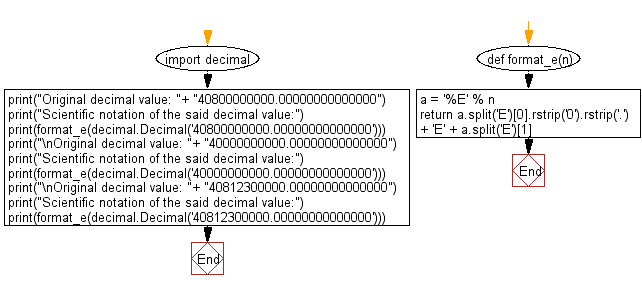﻿ Python: Display a given decimal value in scientific notation - w3resource# Python: Display a given decimal value in scientific notation

## Python module: Exercise-7 with Solution

Write a Python program to display a given decimal value in scientific notation. Use decimal.Decimal

Sample Solution:

Python Code:

``````import decimal
#Source: https://bit.ly/2SfZEtL
def format_e(n):
a = '%E' % n
return a.split('E').rstrip('0').rstrip('.') + 'E' + a.split('E')

print("Original decimal value: "+ "40800000000.00000000000000")
print("Scientific notation of the said decimal value:")
print(format_e(decimal.Decimal('40800000000.00000000000000')))
print("\nOriginal decimal value: "+ "40000000000.00000000000000")
print("Scientific notation of the said decimal value:")
print(format_e(decimal.Decimal('40000000000.00000000000000')))
print("\nOriginal decimal value: "+ "40812300000.00000000000000")
print("Scientific notation of the said decimal value:")
print(format_e(decimal.Decimal('40812300000.00000000000000')))
```
```

Sample Output:

```Original decimal value: 40800000000.00000000000000
Scientific notation of the said decimal value:
4.08E+10

Original decimal value: 40000000000.00000000000000
Scientific notation of the said decimal value:
4E+10

Original decimal value: 40812300000.00000000000000
Scientific notation of the said decimal value:
4.08123E+10
```

Flowchart:## Visualize Python code execution:

The following tool visualize what the computer is doing step-by-step as it executes the said program:

Python Code Editor:

Have another way to solve this solution? Contribute your code (and comments) through Disqus.

What is the difficulty level of this exercise?

Test your Python skills with w3resource's quiz

﻿

## Python: Tips of the Day

For-else construct useful when searched for something and find it:

```# For example assume that I need to search through a list and process each item until a flag item is found and
# then stop processing. If the flag item is missing then an exception needs to be raised.

for i in mylist:
if i == theflag:
break
process(i)
else:
raise ValueError("List argument missing terminal flag.")
```